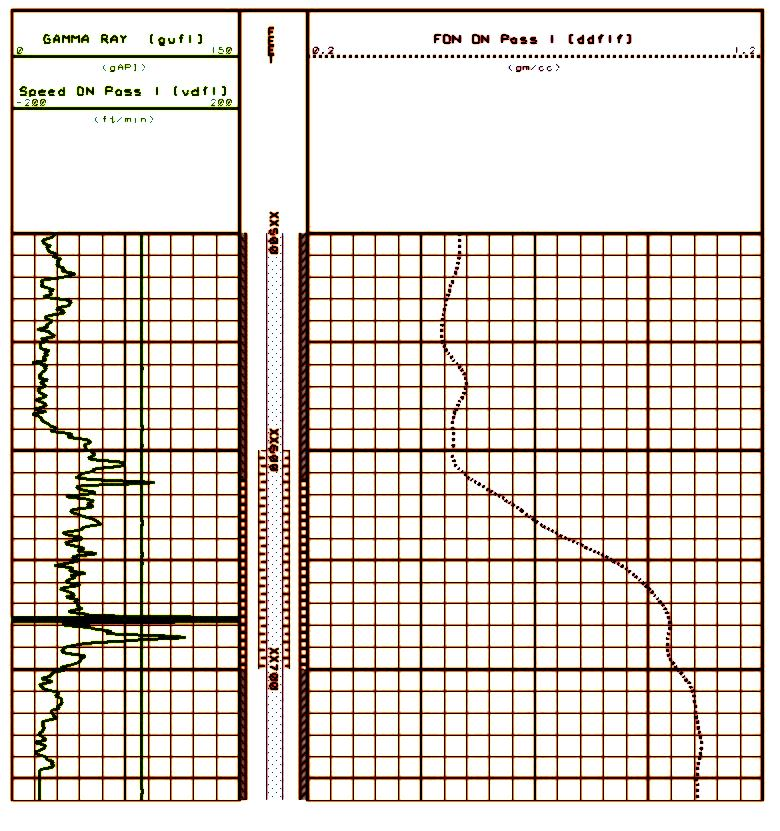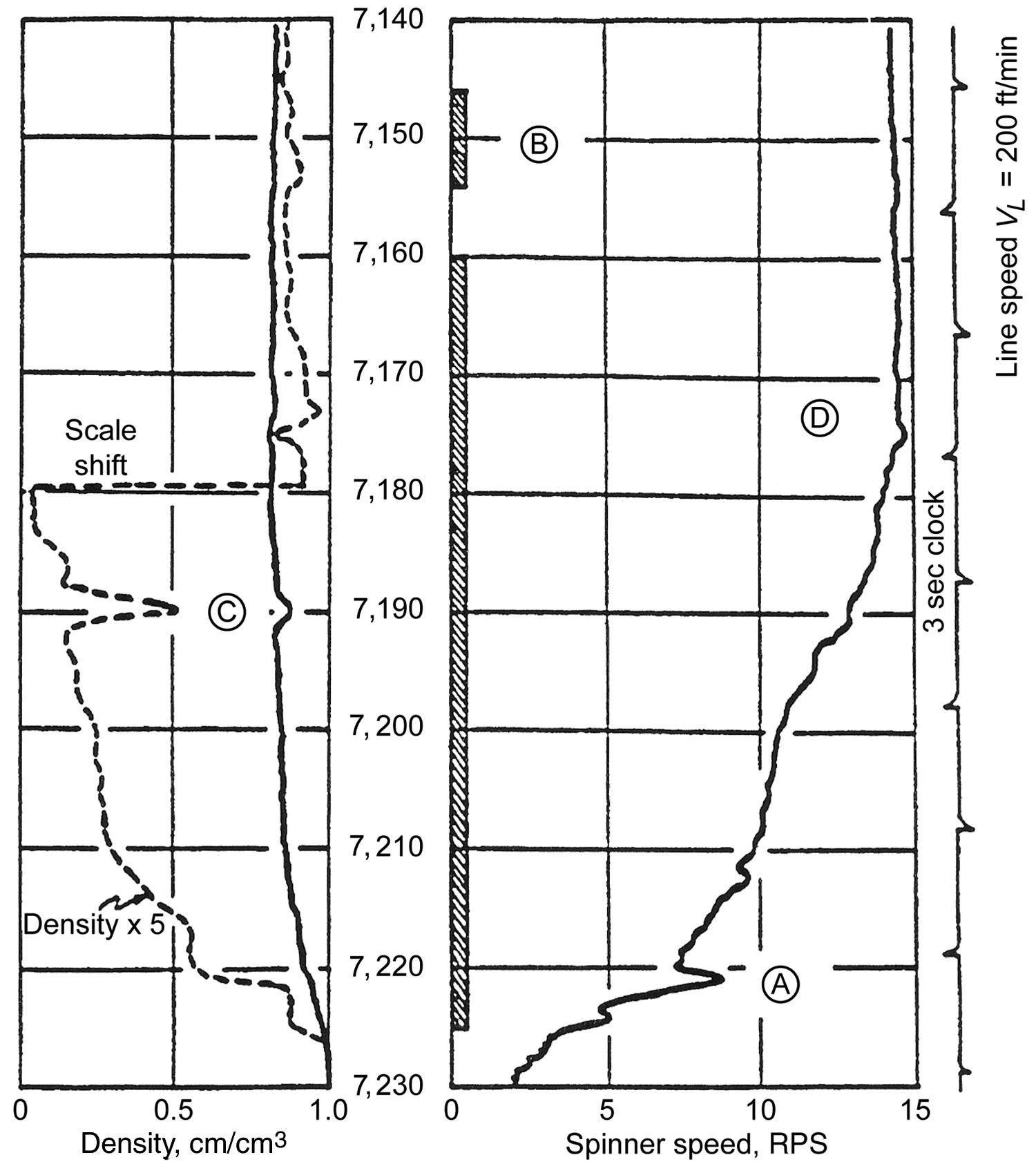FLUID IDENTIFICATION  LOGGING BASICS
The flowmeter tells us how much fluid is moving at any point in a production or injection well, but it tells us nothing about which fluids are present or where each came from. To resolve this, the logging industry has come up with a number of solutions, described later on this page.

### Portions of this article are from the Schlumberger Glossary, with minor edits and additions from other sources such as PetroWiki. The term “holdup” appears often in fluid flow literature. It has nothing to do with gun violence or the price of gasoline at the service station. It really relates to the fraction of the total flow contributed by each fluid phase, oil, gas, and water. In multi-phase flow, “holdup” is the fraction of a particular fluid present in an interval of pipe. Each fluid moves at a different speed due to different gravitational forces (fluid density effect) and other factors (viscosity effect), with the heavier phase moving slower, or being more “held up”, than the lighter phase. The sum of the fluid holdups of the fluids present is unity. The “holdup ratio” is the ratio of the holdups of any two of the fluids. For example, if the water holdup at a particular depth is 0.20, then oil holdup is (1.00 – 0.20) = 0.80 and holdup ratio of oil to water is 0.80 / 0.20 = 4.0. The holdup of a particular fluid is not the same as the proportion of the total flow rate due to that fluid, also known as its “cut”. To determine in-situ flow rates, it is necessary to measure both holdup and velocity of each fluid. A “holdup” log is a record of the fractions of different fluids present versus depth in the borehole. Various techniques are used to measure these fractions. The earliest techniques measured the fluid density, using a gradiomanometer, nuclear fluid density log, or the dielectric properties as in the fluid capacitance log. While these techniques were satisfactory in near-vertical wells with two-phase flow, they were often found to be inadequate in highly deviated and horizontal wells, where flow structures are complex. More recent developments are based on the use of multiple local probes (eg. array capacitance and array spinner imaging tools) to detect bubbles of gas, oil, or water, and on a combination of nuclear techniques usually known as three-phase holdup logs.GRADIOMANOMETER

Introduced in the late 1950s, the gradiomanometer measures the pressure difference between two pressure sensors, placed approximately 2 feet (0.6 m) apart. The pressure difference reflects the average fluid density within that depth interval. The resolution is high, around 0.005 g/cc, but the accuracy can be affected by a friction effect, a kinetic effect, and well deviation. The effect of deviation can be corrected, but the sensitivity to holdup is reduced as the deviation increases until it is zero in a horizontal well.

If we know or can estimate the density of the individual fluid components (oil, gas, water), we can calculate the relative fraction of each fluid present. This allows holdups to be determined, directly in the case of two-phasic flow, and in combination with other measurements for three-phasic flow.

The partitioning equation for a two-phase system is:
1: HoldUp1 = (DENS - DENS2) / (DENS1 - DENS2)
2: HoldUp2 = 1 - HoldUp1
Where:
HoldUp1 = fraction of fluid 1 at this depth
HoldUp2 = fraction of fluid 2 at this depth
DENS = density of fluid mixture (g/cc or kg/m3)
DENS1 = density of fluid 1 (g/cc or kg/m3)
DENS2 = density of fluid 2 (g/cc or kg/m3)

With the well shut in and if there is a sump below the perfs, it often contains formation water so water density can be measured directly by the log. Some distance above this, a direct measurement of oil density can be made. Then during stable production, the complete log for water holdup can be run.

Numerical Example
Assume
DENS1 = downhole water density = 1.110 g/cc
DENS2 = downhole oil density = 0.710 g/cc
Composite fluid density = 0.810 g/cc

Water Holdup  = (0.810 - 0.710)/(1.110 - 0.710) = 0.100 / 0.400 = 0.25  (or 25%)
Oil Holdup  = (1.0 - 0.25) = 0.75  (or 75%)FLUID CAPACITANCE LOGS
This log provides a record of the capability of the fluid passing through a sensor to store electrical charge. Since water has a high dielectric constant, and hence capacitance, the log can distinguish water from oil or gas. The fluid capacitance log can therefore identify water and be scaled in terms of water holdup. However the relation between capacitance and holdup depends strongly on whether the water is the continuous phase, complicating quantitative evaluation.

The log was introduced in the 1960s as the so-called holdup meter. It was mainly used in three-phase flow, or when fluid-density measurements were insufficiently sensitive to water at low holdup, or with heavy oils. Since the late 1980s, other holdup measurements have been preferred.NUCLEAR FLUID DENSITY LOG
Tool names used by the many service providers are confusing or misleading. Focused gamma ray and unfocused gamma ray logs, for example, do not record a gamma ray log, but they do use a chemical gamma ray source to create a simple uncompensated density log with a very small range of investigation.

These logs measure the density of fluids in a completed well, using a radioactive source of gamma rays and a detector. A cesium 137 or americium 241 source is used to induce Compton scattering, as in the openhole density log, except that the device is unfocused. The count rate at the detector depends primarily on the density of the fluids in the well.

In unfocused  devices, the source and detector are situated so that the gamma rays pass outside the tool. The results reflect an average density of all the fluids within the well at that depth. In smaller casings, some formation signal may contaminate the measurement. This type of density log has been used to assess the quality of gravel pack completions.

On some devices, the fluids pass through an open space in the body of the tool within which the measurement is made. The results reflect the density of the fluids passing through the tool. Some people call this type of tool a focused fluid density log since the gamma rays are focused on the wellbore fluid and are not influenced by anything outside the tool body.

Most fluid density logs are scaled in density units (g/cc) but some may have a counts per second (cps) scale. Holdup is calculated as explained for the gradiomanometer,Example of a fluid density log using a chemical gamma ray source. Density scale is 0.6 to 1.1 g/cc. Both water and oil densities can be read directly from the log. A natural gamma ray log is in Track1 for correlation.

Compared with a gradiomanometer, the nuclear fluid density log is a less direct measurement of density, and has a statistical uncertainty and less resolution, but it is not affected by well deviation, friction, or kinetic effects.Fluid density (track 1) and spinner survey (tracks 2 and 3). Well flowing 10,000 bpd. Density shows water density in sump below perfs, oil density over perf interval. Spinner shows increasing cumulative production over lower half of perfs. The upper half of the perfs may have been ineffective or the reservoir quality is so poor that no flow can be expected. A competent forensic  petrophysical analysis could answer this question and a workover initiated if warranted. Amplified fluid density curve shows slight decrease up to 7160 feet indicating some water in the oil below this depth - this is the "water holdup".

Page Views ---- Since 15 Jul 2020
Copyright 2023 by Accessible Petrophysics Ltd.
CPH Logo, "CPH", "CPH Gold Member", "CPH Platinum Member", "Crain's Rules", "Meta/Log", "Computer-Ready-Math", "Petro/Fusion Scripts" are Trademarks of the Author